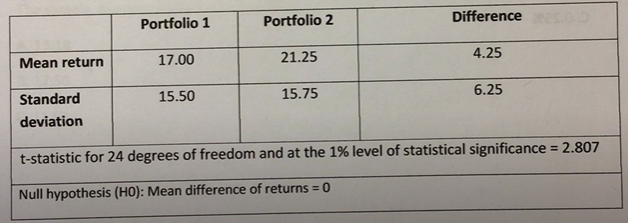## Free CFA Level 1 Sample Quiz 50 Questions Answer Keys AMBIPi

Hi CFA Aspirants, welcome to AKVTutorials. Are you preparing for CFA Level 1, 2, 3 exams for making a career in CFA (Charted Financial Analyst). According to CFA Wikipedia, CFA The Chartered Financial Analyst (CFA) program is a postgraduate professional certification offered internationally by the American-based CFA Institute. A candidate who successfully completes the program and meets other professional requirements is awarded the “CFA charter” and becomes a “CFA charter holder”. Therefore, you need CFA Study Notes and Free CFA Level 1 Sample Quiz 50 Questions Answer Keys AMBIPi.

In this article, you will get Free CFA Level 1 Mock Exam Practice Questions.

## Free CFA Level 1 Mock Practice Exam Questions Bank

Free CFA Level 1 Practice Question No: 491:

The following information is available for a portfolio:The return on the portfolio is closest to:

Option A : 10.0%

Option B : 8.2%

Option C : 10.8%

Option C: 10.8%

CFA Level 1 Exam Question No: 492:

A sample of 240 managed portfolios has a mean annual return of 0.11 and a standard deviation of returns of 0.23. The standard error of the sample mean is closest  to:

Option A: 0.00096

Option B: 0.00710

Option C: 0.01485

Option C: 0.01485

Free CFA Level 1 Mock Exam Question No: 493:

A two-tailed t-test of the hypothesis that the population mean differs from zero has a p-value of 0.0275. Using a significance level of 5%, the most appropriate conclusion is:

Option A: to accept the null hypothesis

Option B: to reject the null hypothesis

Option C: that the chosen significance level is too high

Option B: to reject the null hypothesis

CFA Level 1 Free Practice Question No: 494:

Monte Carlo simulation is best described as:

Option A: a restrictive form of scenario analysis

Option B: providing a distribution of possible solutions to complex functions

Option C: an approach to backtest data

Option B : providing a distribution of possible solutions to complex functions

Free CFA Practice Question No: 495:

The belief that trends and patterns tend to repeat themselves and are, therefore, somewhat predictable best describes:

Option A: arbitrage pricing theory

Option B: technical analysis

Option C: weak-form efficiency

Option B : technical analysis

CFA Level 1 Sample Question No: 496:

Which of the following most accurately describes a distribution that is more peaked than normal?

Option A: Mesokurtotic

Option B: Platykurtotic

Option C: Leptokurtotic

Option C : Leptokurtotic

Free CFA Level 1 Quiz Question NO: 497:

The null hypothesis is most likely to be rejected when the p-value of the test statistic:

Option A: exceeds a specified level of significance

Option B: is negative

Option C: falls below a specified level of significance

Option C : falls below a specified level of significance

Free CFA Level 1 Quiz Question NO: 498:

Using the following sample results drawn as 25 paired observations from their underlying distributions, test whether the mean returns of the two portfolios differ from each other at the 1% level of statistical significance. Assume the underlying distributions of returns for each portfolio are normal and that their population variances are not known.Based on the paired comparisons test of the two portfolios, the most appropriate conclusion is that но should be:

Option A: accepted because the computed test statistic is less than 2.807

Option B: rejected because the computed test statistic exceeds 2.807

Option C: accepted because the computed test statistic exceeds 2.807

Option B: rejected because the computed test statistic exceeds 2.807

Free CFA Practice Question No: 499:

Over the past four years, a portfolio experienced returns of -8%, 4%, 17%, and -12%. The geometric mean return of the portfolio over the four-year period is closest to:

Option A: 0.99%

Option B: -0.37%

Option C: 0.25%

Option B : -0.37%

CFA Mock Exam Free Question No: 500:

Based on historical returns, a portfolio has a Sharpe ratio of 2.0. If the mean return to the portfolio is 20%, and the mean return to a risk-free asset is 4%, the standard deviation of return of the portfolio is closest to:

Option A: 12%

Option B: 8%

Option C: 10%